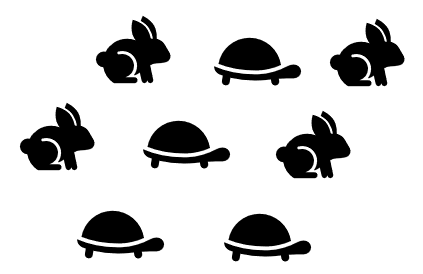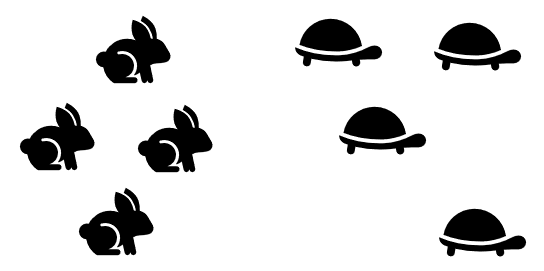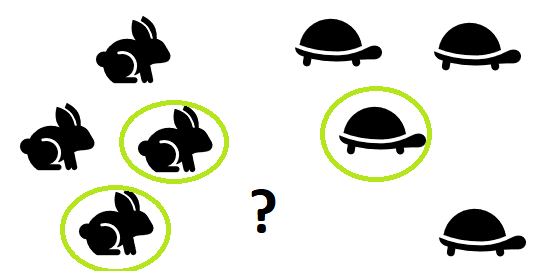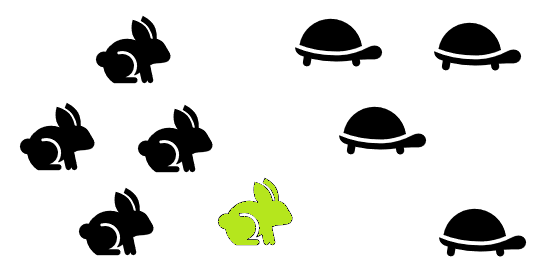# k-NN (k-Nearest Neighbor): Overview, Simple Example

Share on

Nearest Neighbor matching > k-NN (k-Nearest Neighbor)

K-nn (k-Nearest Neighbor) is a non-parametric classification and regression technique. The basic idea is that you input a known data set, add an unknown, and the algorithm will tell you to which class that unknown data point belongs. The unknown is classified by a simple neighborly vote, where the class of close neighbors “wins.” It’s most popular use is for predictive decision making. For example:

• Will a customer default on a loan, or not?
• Is the business going to make a profit?
• Should we expand into a certain market segment?

The following simple example shows how k-nn works.

## 1. Take a dataset with known categories

In this initial step, you’re just collecting the unsorted, raw data. In this example, the data is clearly categorized with hares and tortoises.## 2. Cluster the data

You’ve got a few choices in this step; How you cluster the data is up to you. (e.g. with PCA or another clustering method).## 4. Find the “k”

Perhaps the most challenging step is finding a k that’s “just right”. The square root of n (the number of items in the data set) is an easy place to start.

• √(n)
• = √(8)
• = 2.82
• = ≅ 3

Although the square root of n is simple, it isn’t the most accurate method. Ideally you should use a training set (i.e. a nicely categorized set) to find a “k” that works for your data. Remove a few categorized data points and make them “unknowns”, testing a few values for k to see what works. An elbow method can work well, where you find an optimal k based on lowest error rates. For a brief look at the elbow method, see: Determining number of clusters in one picture.

## 5. Locate the “k” nearest neighbors

For this example, I just used the visual to locate the nearest neighbors.## 6. Classify the new point

The new point is classified by a majority vote. If most of your neighbors are turtles, odds are that you’re also a turtle. In this case, two out of three of the unknown’s neighbors are hares so the new point is classified as a hare.